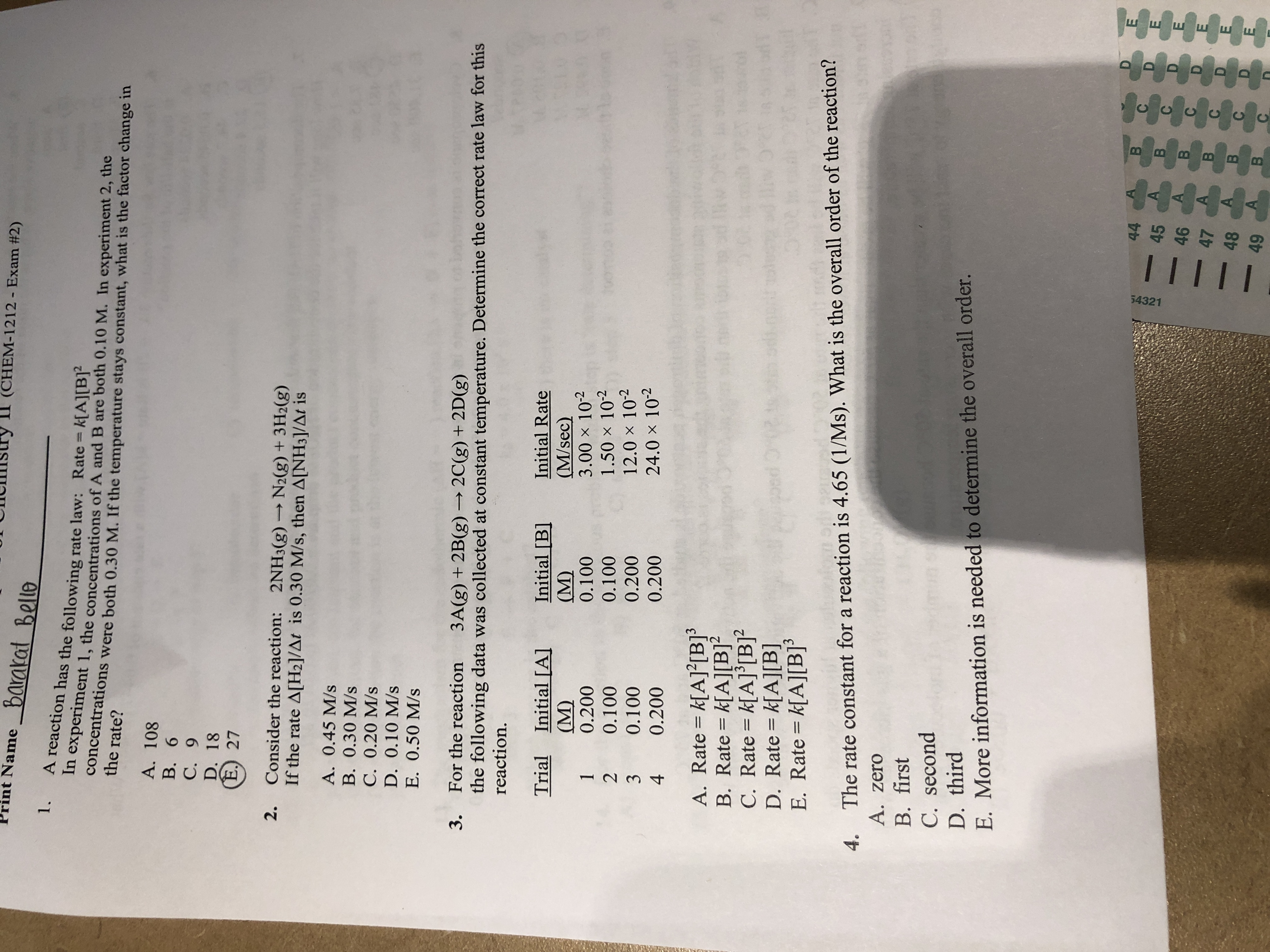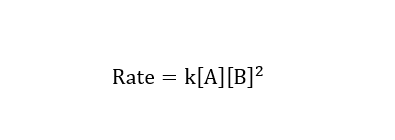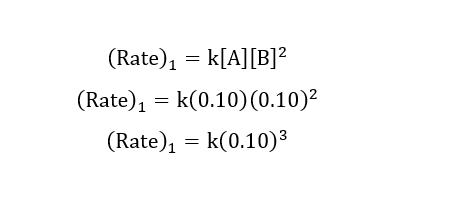# int Name barakat BelloII (CHEM-1212 - Exam #2)1.A reaction has the following rate law: Rate k[A][B]²In experiment 1, the concentrations of A and B are both 0.10 M. In experiment 2, theConcentrations were both 0.30 M. If the temperature stays constant, what is the factor change in%3Dthe rate?A. 108B. 6C. 9D. 18E.) 27Consider the reaction: 2NH3(g) →N2(g) + 3H2(g)If the rate A[H2]/At is 0.30 M/s, then A[NH3]/At isA. 0.45 M/sB. 0.30 M/sC. 0.20 M/sD. 0.10 M/sE. 0.50 M/s3. For the reaction3A(g) + 2B(g) → 2C(g) + 2D(g)the following data was collected at constant temperature. Determine the correct rate law for thisreaction.Initial Rate(M/sec)3.00 x 1021.50 x 10212.0 x 10224.0 x 10-2Initial [A](M)0.200Initial [B](M)0.100Trial0.1000.1000.1000.2000.2000.200A. Rate = k[A]°[B]³B. Rate = k[A][B]?C. Rate = k[A]°[B]?D. Rate = k[A][B]E. Rate = k[A][B]³%3D%3D4. The rate constant for a reaction is 4.65 (1/Ms). What is the overall order of the reaction?A. zeroB. firstC. secondD. thirdE. More information is needed to determine the overall order.E.4445A.46474849B.шшш шЕBBB 3115432112342.

Question
1 viewshelp_outlineImage Transcriptioncloseint Name barakat Bello II (CHEM-1212 - Exam #2) 1. A reaction has the following rate law: Rate k[A][B]² In experiment 1, the concentrations of A and B are both 0.10 M. In experiment 2, the Concentrations were both 0.30 M. If the temperature stays constant, what is the factor change in %3D the rate? A. 108 B. 6 C. 9 D. 18 E.) 27 Consider the reaction: 2NH3(g) →N2(g) + 3H2(g) If the rate A[H2]/At is 0.30 M/s, then A[NH3]/At is A. 0.45 M/s B. 0.30 M/s C. 0.20 M/s D. 0.10 M/s E. 0.50 M/s 3. For the reaction 3A(g) + 2B(g) → 2C(g) + 2D(g) the following data was collected at constant temperature. Determine the correct rate law for this reaction. Initial Rate (M/sec) 3.00 x 102 1.50 x 102 12.0 x 102 24.0 x 10-2 Initial [A] (M) 0.200 Initial [B] (M) 0.100 Trial 0.100 0.100 0.100 0.200 0.200 0.200 A. Rate = k[A]°[B]³ B. Rate = k[A][B]? C. Rate = k[A]°[B]? D. Rate = k[A][B] E. Rate = k[A][B]³ %3D %3D 4. The rate constant for a reaction is 4.65 (1/Ms). What is the overall order of the reaction? A. zero B. first C. second D. third E. More information is needed to determine the overall order. E. 44 45 A. 46 47 48 49 B. шшш шЕ BBB 3 11 54321 1234 2. fullscreen
check_circle

Step 1

Hi there, as there are multiple questions posted. We are answering the first question. If you need further questions to be answered please repost the question with specific question number.

Step 2

Given rate law:Step 3

Rate of reaction of experiment-1:...

### Want to see the full answer?

See Solution

#### Want to see this answer and more?

Solutions are written by subject experts who are available 24/7. Questions are typically answered within 1 hour.*

See Solution
*Response times may vary by subject and question.
Tagged in